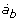# Table 2 Protein metabolism - equations.

State variables
a(t) = (a a , a p , a b , p a )T
a a = amino acids in the free AA pool
a p = amino acids channeled to protein synthesis
a b = amino acids channeled to catabolism
p a = tissue protein given by its amino acid composition
u aa = 20 amino acids that are recieved from the feed (input variable)
State equations= -k aa a a - U ps k p a a + u aa k aa = 1/s= U ps k p a a - v s (t)a p + f p v d (t)p a k p = 1/s= k aa a a + f t v d (t)p a - ab, cat= v s (t)a p - v d (t)p a
1. State vector a(t) and equations for the protein metabolism submodel illustrated in Figure 9.